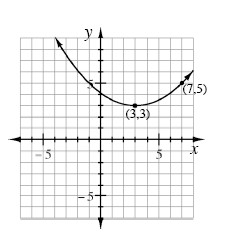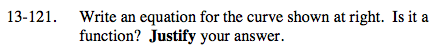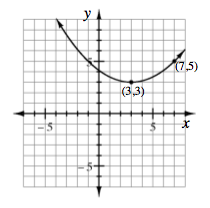### Home > A2C > Chapter 13 > Lesson 13.2.2 > Problem13-121

13-121.

Write an equation for the curve shown below. Is it a function? Justify your answer. Homework Help ✎Use the vertex to write an equation
for a parabola in graphing form.

y = a(x − 3)2 + 3

Use the other given point to solve for a.$y=\frac{1}{8}(x-3)^{2}+3$